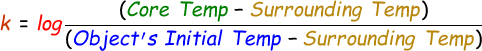# Newton's Law of Cooling Calculator

The newton's law of cooling explains that the rate of change of object's temperature is directly proportionals to the own variations in temperature and the surrounding temperature.

Formula to calculate newton's law of cooling is given by:where,
T(t) = Object's temperature at time t
Ts = Surrounding temperature
To = Object's initial temperature
T = Core Temperature
t = time

For the above equation, k can be calculated like this:In our online newton's law of cooling calculator below, enter the surrounding temperature, object's initial temperature, core temperature and time in the input fields and then click calculate to find the answer.

 Surrounding Temperature (Ts): Object's Initial Temperature ((To)): Core Temperature (T): Time (t): Object's temperature at time t (T(t)):

Latest Calculator Release

Average Acceleration Calculator

Average acceleration is the object's change in speed for a specific given time period. ...

Free Fall Calculator

When an object falls into the ground due to planet's own gravitational force is known a...

Torque Calculator

Torque is nothing but a rotational force. In other words, the amount of force applied t...

Average Force Calculator

Average force can be explained as the amount of force exerted by the body moving at giv...

Angular Displacement Calculator

Angular displacement is the angle at which an object moves on a circular path. It is de...